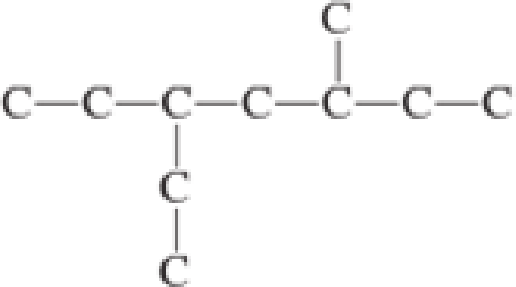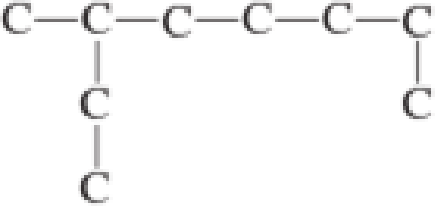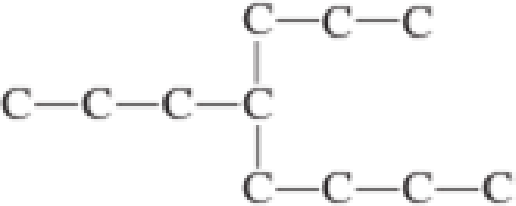Chapter 1, Problem 1.47EP### Organic And Biological Chemistry

7th Edition
STOKER + 1 other
ISBN: 9781305081079

#### Solutions

Chapter
Section### Organic And Biological Chemistry

7th Edition
STOKER + 1 other
ISBN: 9781305081079
Textbook Problem

# The first step in naming an alkane is to identify the longest continuous chain of carbon atoms. For each of the following skeletal structural formulas, how many carbon atoms are present in the longest continuous chain?a.b.c.d.(a)

Interpretation Introduction

Interpretation:

The total number of carbon atoms that is present in the longest continuous chain has to be found for the given skeletal structural formulas.

Concept Introduction:

Any organic molecule can be named by using certain rules given by IUPAC (International Union for Pure and applied chemistry).  IUPAC name consists of three parts in major namely Prefix suffix and root word.

Prefix represents the substituent present in the molecule and its position in the root name.

Suffix denotes the presence of functional group if any in the molecule.  It can be an alkene, alkyne, alcohol, carboxylic acid, alcohol etc.

Root word represents the longest continuous carbon skeleton of the organic molecule.

To name an alkane the first step is to found out the longest continuous chain.  The other carbon atoms that are present apart from the longest carbon chain is the substituents.

Explanation

Given skeletal structural formula is,

To find the longest continuous chain, the various possibilities have to be explored.  This can be done as shown below,

(b)

Interpretation Introduction

Interpretation:

The total number of carbon atoms that is present in the longest continuous chain has to be found for the given skeletal structural formulas.

Concept Introduction:

Any organic molecule can be named by using certain rules given by IUPAC (International Union for Pure and applied chemistry).  IUPAC name consists of three parts in major namely Prefix suffix and root word.

Prefix represents the substituent present in the molecule and its position in the root name.

Suffix denotes the presence of functional group if any in the molecule.  It can be an alkene, alkyne, alcohol, carboxylic acid, alcohol etc.

Root word represents the longest continuous carbon skeleton of the organic molecule.

To name an alkane the first step is to found out the longest continuous chain.  The other carbon atoms that are present apart from the longest carbon chain is the substituents.

(c)

Interpretation Introduction

Interpretation:

The total number of carbon atoms that is present in the longest continuous chain has to be found for the given skeletal structural formulas.

Concept Introduction:

Any organic molecule can be named by using certain rules given by IUPAC (International Union for Pure and applied chemistry).  IUPAC name consists of three parts in major namely Prefix suffix and root word.

Prefix represents the substituent present in the molecule and its position in the root name.

Suffix denotes the presence of functional group if any in the molecule.  It can be an alkene, alkyne, alcohol, carboxylic acid, alcohol etc.

Root word represents the longest continuous carbon skeleton of the organic molecule.

To name an alkane the first step is to found out the longest continuous chain.  The other carbon atoms that are present apart from the longest carbon chain is the substituents.

(d)

Interpretation Introduction

Interpretation:

The total number of carbon atoms that is present in the longest continuous chain has to be found for the given skeletal structural formulas.

Concept Introduction:

Any organic molecule can be named by using certain rules given by IUPAC (International Union for Pure and applied chemistry).  IUPAC name consists of three parts in major namely Prefix suffix and root word.

Prefix represents the substituent present in the molecule and its position in the root name.

Suffix denotes the presence of functional group if any in the molecule.  It can be an alkene, alkyne, alcohol, carboxylic acid, alcohol etc.

Root word represents the longest continuous carbon skeleton of the organic molecule.

To name an alkane the first step is to found out the longest continuous chain.  The other carbon atoms that are present apart from the longest carbon chain is the substituents.

### Still sussing out bartleby?

Check out a sample textbook solution.

See a sample solution

#### The Solution to Your Study Problems

Bartleby provides explanations to thousands of textbook problems written by our experts, many with advanced degrees!

Get Started